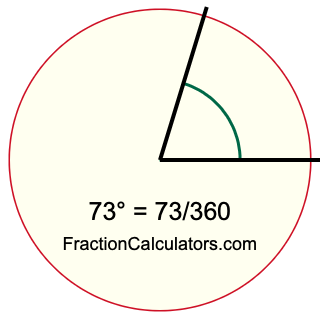What fraction of a circle is 73 degrees?Here we will explain, calculate, and show you what fraction of a circle 73 degrees is. More specifically, if you draw two lines from the center of a circle, creating 73 degrees, what will the area between the lines be as a fraction of the whole circle?

A full turn on a circle is 360 degrees. Therefore, to calculate what fraction of a circle any number of degrees is, you can simply put the number of degrees over 360.

When we put 73 over 360 and simplify if necessary, we get the answer to "What fraction of a circle is 73 degrees?" as follows:

degrees/360 = fraction
73/360 = 73/360
73 degrees = 73/360

Below is an illustration showing you what 73 degrees and 73/360 of a circle looks like.To create the illustration above showing you 73 degrees, we first drew a circle and then drew two lines from the center, separated by 73 degrees. The slice that the two lines create inside the circle is 73/360 of the circle.

Degrees to Fraction of a Circle Calculator
Here you can convert another degree to a fraction of a circle.

What fraction of a circle is  degrees?

What fraction of a circle is 74 degrees?
Here is another degree we have converted to a fraction for you.